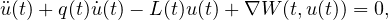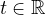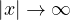Electron. J. Differential Equations, Vol. 2019 (2019), No. 43, pp. 1-17.

### Fast homoclinic solutions for damped vibration systems with subquadratic and asymptotically quadratic potentials Yiwei Ye

Abstract:where L(t) is uniformly positive definite for all, and W(t,x) is either subquadratic or asymptotically quadratic in x as. Based on the minimax method in critical point theory, we prove the existence and multiplicity of fast homoclinic solutions for the above problem.Yiwei Ye School of Mathematical Science Chongqing Normal University Chongqing 401331, China email: yeyiwei2011@126.com